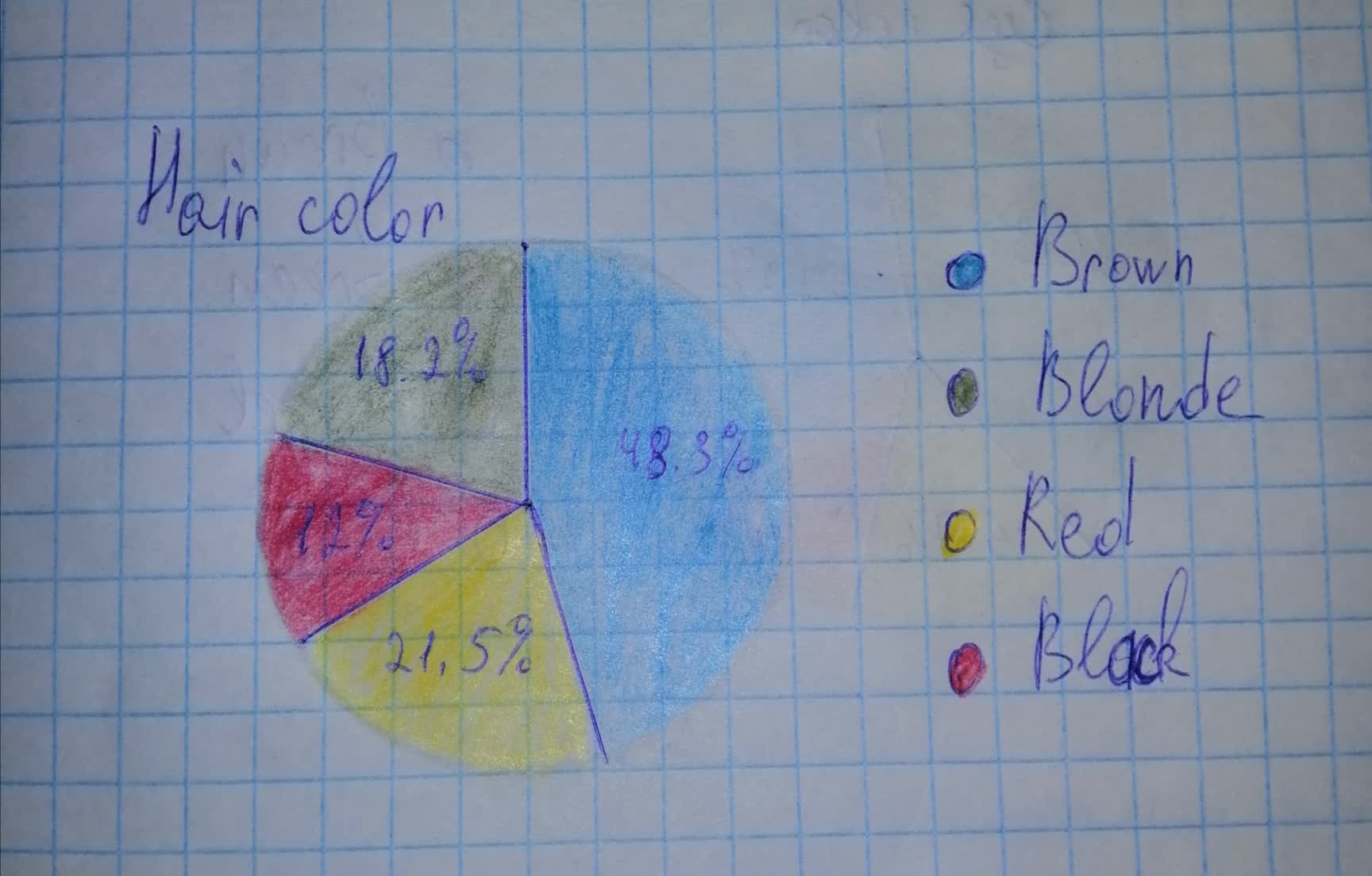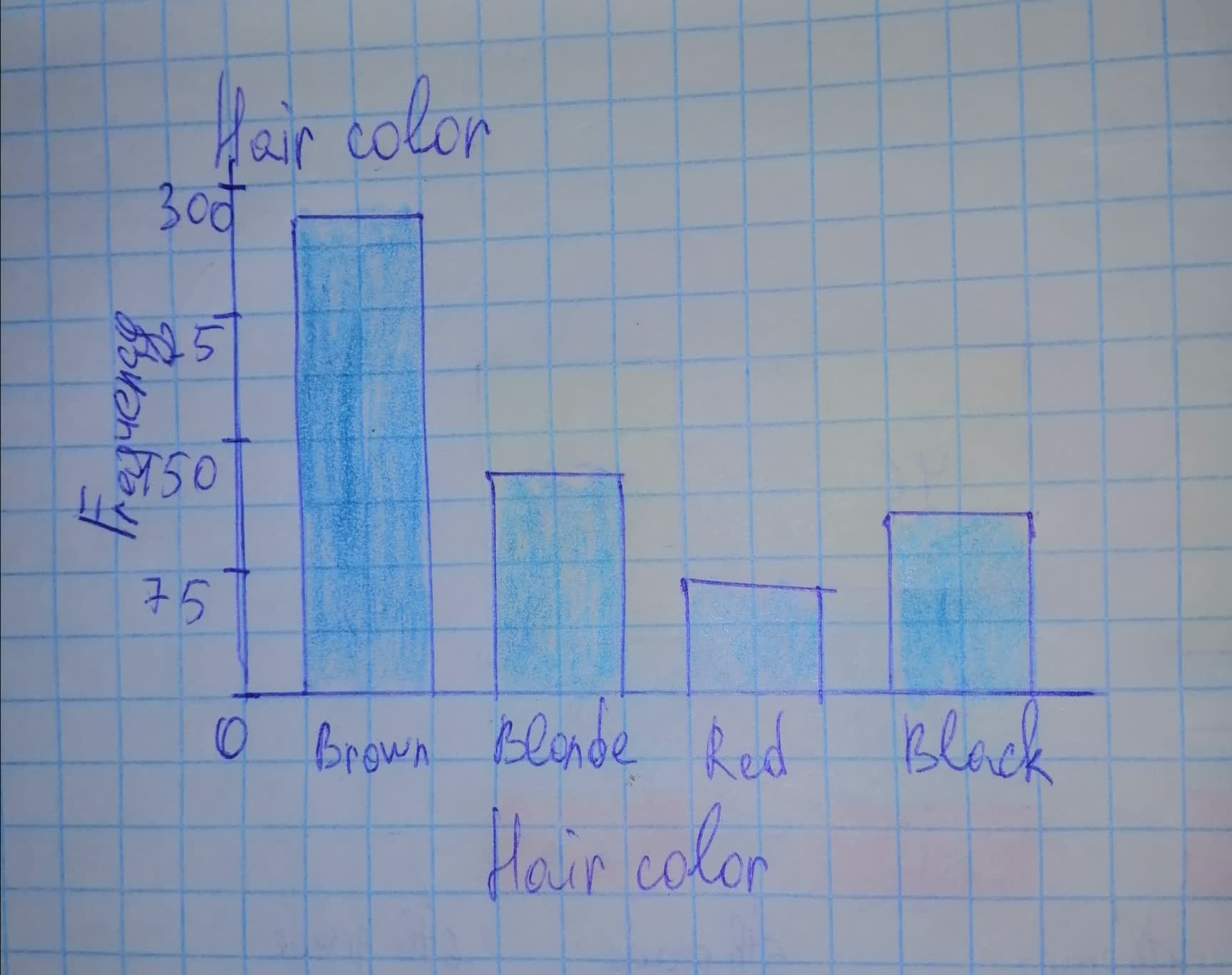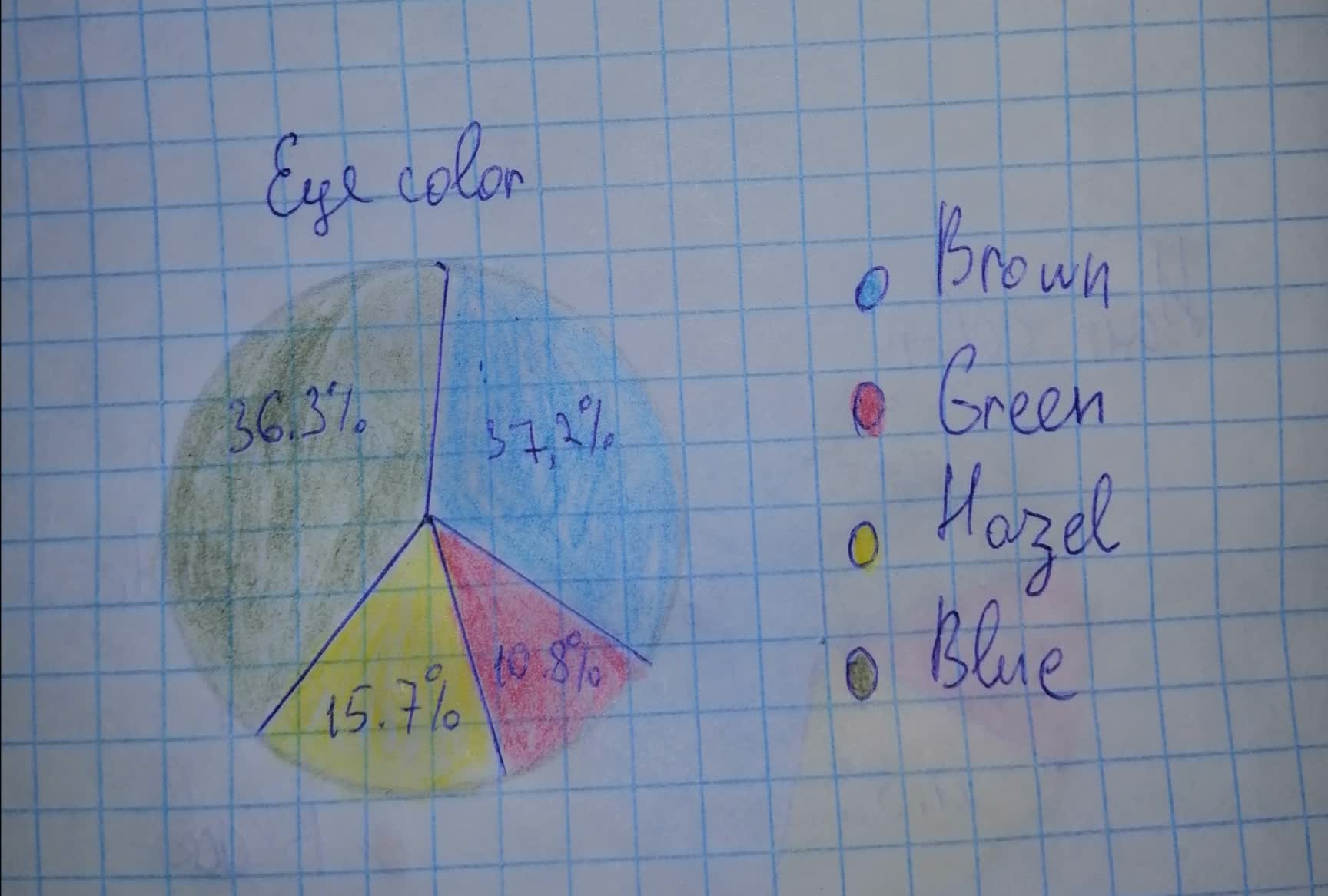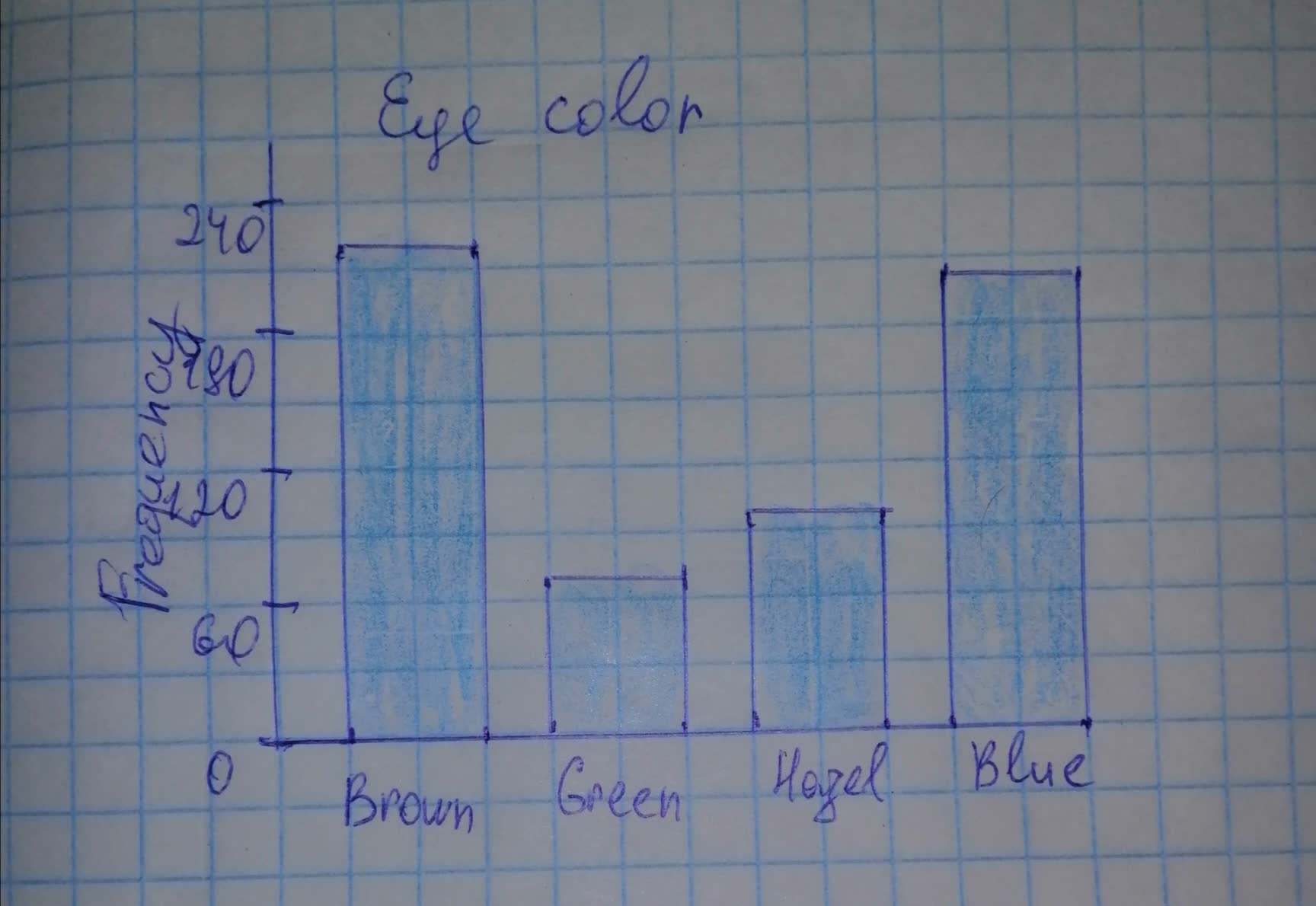# In the article "Graphical Display of Two-Way Contingency Tables", R. Snee presenMaaghu 2021-11-21 Answered
In the article "Graphical Display of Two-Way Contingency Tables", R. Snee presented data on hair color and eye color among 592 students in an elementary statistics course at the University of Delaware. Raw data for that information are presented on the WeissStats site. Use the technology of your choice to do the following tZSKs, and interpret your results.
a) Obtain both a frequency distribution and a relative-frequency distribution for the hair-color data.
b) Get a pie chart of the hair-color data.
c) Determine a bar chart of the hair-color data.
d) Repeat parts (a)-(c) for the eye-color data.

• Questions are typically answered in as fast as 30 minutes

### Plainmath recommends

• Get a detailed answer even on the hardest topics.
• Ask an expert for a step-by-step guidance to learn to do it yourself.Nicole Keller

Step 1
Solution:
a) The frequency is the number of times that the value occurs in the data set.
The relative frequency is the frequency divided by the total frequency.
$\begin{array}{|c|c|}\hline \text{Value} & \text{Frequency} & & \text{Relative frequency} & & \\ \hline \text{Brown} & 286 & & \frac{286}{592} & = & 0.4831 \\ \hline \text{Blonde} & 127 & & \frac{127}{592} & = & 0.2145 \\ \hline \text{Red} & 71 & & \frac{71}{592} & = & 0.1199 \\ \hline \text{Black} & 108 & & \frac{108}{592} & = & 0.1824 \\ \hline \text{Total} & 592 & & & & 1 \\ \hline \end{array}$
Step 2
b) Pie chart
Draw a circle
The slice size (central angle of the circle) is the product of 360 degrees and the percent of values in the category.
Draw the slices for each category.Step 3
c) Bar chart
The width of the bars has to be the same and the height has to be equal to the frequency.Step 4
d) The frequency is the number of times that the value occurs in the data set.
The relative frequaency is the frequency divided by the total frequency.
$\begin{array}{|c|c|}\hline \text{Value} & \text{Frequency} & & \text{Relative frequency} & & \\ \hline \text{Brown} & 220 & & \frac{220}{592} & = & 0.3716 \\ \hline \text{Blonde} & 64 & & \frac{64}{592} & = & 0.1081 \\ \hline \text{Red} & 93 & & \frac{93}{592} & = & 0.1571 \\ \hline \text{Black} & 215 & & \frac{215}{592} & = & 0.3632 \\ \hline \text{Total} & 592 & & & & 1 \\ \hline \end{array}$
Step 5
Pie chart
Draw a circle
The slice size (central angle of the circle) is the product of 360 degrees and the percent of values in the category.
Draw the slices for each category.Step 6
Bar chart
The width of the bars has to be the same and the height has to be equal to the frequency.